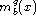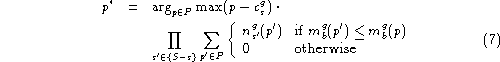Next: Agents with Two-level Models Up: Agents with One-level Models Previous: Buyers with one-level models.#### Sellers with one-level models.

Each seller will try to predict what bid the other sellers will submit (based solely on what they have bid in the past), and what bid the buyer will likely pick. A complete implementation would require the seller to remember past combinations of buyers, bids and results (i.e. who was buying, who bid what, and who won). However, it is unrealistic to expect a seller to remember all this since there are at leastpossible combinations.

We believe, however, that the seller's one-level behavior can be approximated by having him remember the last N prices accepted by each buyer b for each good g, and form a probability density function, which returns the probability that b will accept(pick) price p for good g. Similarly, the seller remembers other sellers' last N bids for good g and forms, which gives the probability that s will bid y for good g. The seller s can now determine which bid maximizes his expected profits.Note that this function also does a small amount of approximation by assuming that s wins whenever there is a tie. The function calculates the best bid by determining, for each possible bid, the product of the profit and the probability that the agent will get that profit. Since the profit for lost bids is 0, we only need to consider the cases where s wins. The probability that s will win can then be found by calculating the product of the probabilities that his bid will beat the bids of each of the other sellers.

Jose M. Vidal
jmvidal@umich.edu
Wed Aug 21 07:12:37 EDT 1996